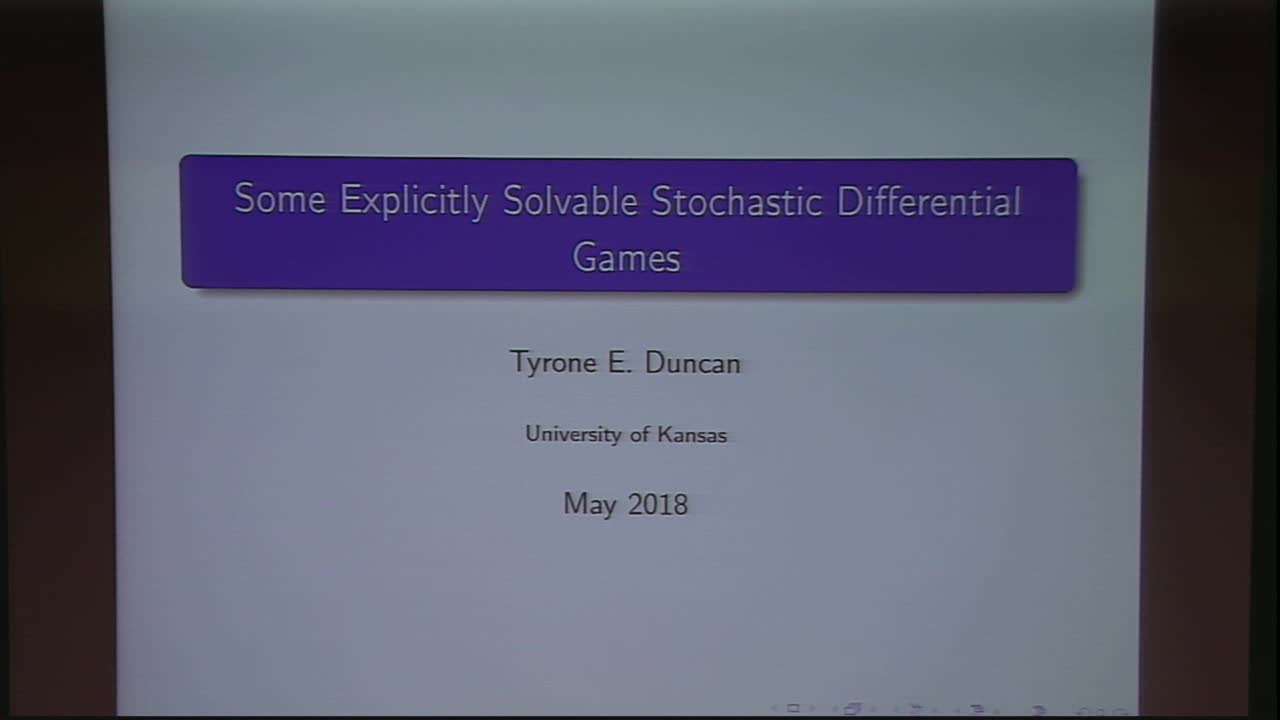## Some Explicitly Solvable Stochastic Differential Games

May 11, 2018
IMA
Presenters: Tyrone Duncan

Watch Video

#### Abstract

Stochastic differential games have been used as models for a wide variety of physical systems. These games are a natural evolution from some stochastic control problems. Two well known methods to find optimal control strategies for a stochastic differential game are solving Hamilton-Jacobi-Isaacs equations which are nonlinear partial differential equations or solving backward stochastic differential equations. Both of these approaches are often difficult to solve for explicit optimal control strategies. A direct method has been used to obtain explicit solutions for a variety of stochastic differential games. This method does not require solving nonlinear partial differential equations or backward stochastic differential equations. Some stochastic differential games are described where this direct method provides explicit optimal strategies. These stochastic differential games include linear stochastic differential games with quadratic or risk sensitive exponential quadratic payoffs, some nonlinear stochastic differential games that evolve in symmetric spaces, and some linear stochastic differential games with quadratic payoffs and state dependent fractional Brownian motion noise. The method can also be used to solve some games described by infinite dimensional systems that model stochastic partial differential equations.